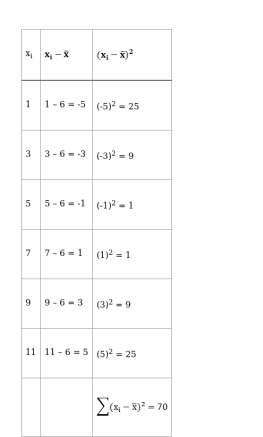Deepak Scored 45->99%ile with Bounce Back Crack Course. You can do it too!

# Find the mean, variance and standard deviation for the first six odd natural numbers

Question:

Find the mean, variance and standard deviation for the first six odd natural numbers

Solution:

Odd natural numbers = 1, 3, 5, 7, 9, …

First Six Odd Natural Numbers = 1, 3, 5, 7, 9, 11

To find: MEAN

We know that

$\operatorname{Mean}(\overline{\mathrm{x}})=\frac{\text { Sum of observations }}{\text { Total number of observations }}$

$=\frac{1+3+5+7+9+11}{6}$

$=\frac{36}{6}$

$\overline{\mathrm{x}}=6$

To find: VARIANCEVariance, $\sigma^{2}=\frac{\sum\left(\mathrm{x}_{\mathrm{i}}-\overline{\mathrm{x}}\right)^{2}}{\mathrm{n}}$

$=\frac{70}{6}$

$=11.67$

To find: STANDARD DEVIATION

Standard Deviation $(\sigma)=\sqrt{\text { Variance }}$

$=\sqrt{11.67}$

$=3.41$